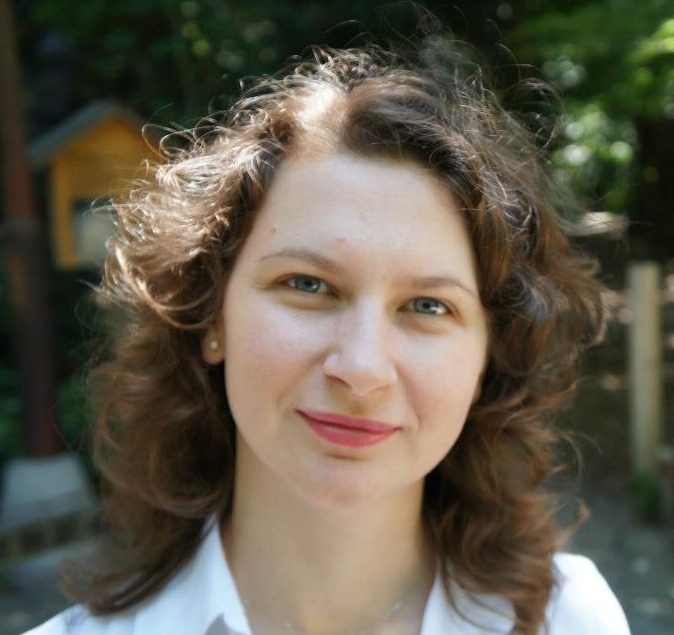Oana Pocovnicu Associate Professor Department of Mathematics at Heriot-Watt University Maxwell Institute for Mathematical Sciences, Edinburgh Mailing address: School of Mathematical & Computer Sciences Heriot-Watt University Edinburgh EH14 4AS, UK Office: Room F.14, Colin Maclaurin Building Telephone: +44 (0)131 451 4371 E-mail: o.pocovnicuhw.ac.uk

Brief CV         Publications           Talks        Slides of some talks          Teaching       Administrative roles       Useful links

Research interests: Mathematical analysis of nonlinear partial differential equations (PDEs):

• Hyperbolic/dispersive semilinear Hamiltonian PDEs (nonlinear wave & Schrödinger equations),
• Completely integrable nonlinear PDEs (Szegő equation),
• Quasilinear PDEs (Euler-Poisson system).
• In particular, study of global-in-time behavior of solutions: global well-posedness in the deterministic and probabilistic settings, scattering, growth of high Sobolev norms related to wave turbulence, existence and stability of solitons, soliton resolution. Tools from: harmonic analysis, completely integrable Hamiltonian equations, complex analysis, spectral theory, mathematical physics, and probability.

Ph.D. opportunities: The Maxwell Institute Graduate School (MIGS)